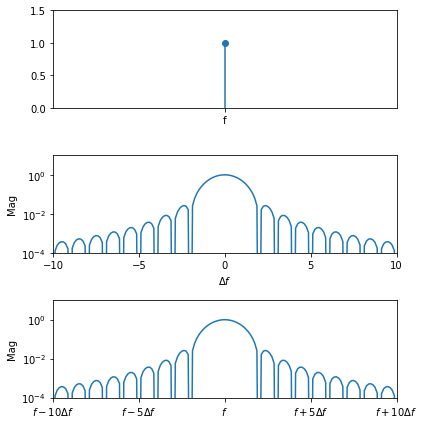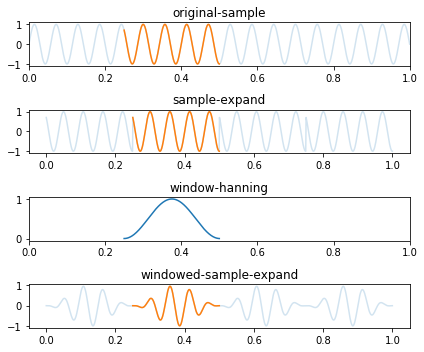x_{win}(n) = x(n) * win(n)

– 矩形窗
– 汉宁窗
– 平顶窗
– Blackman-Harris
– Kaiser-Bessel

### 矩形窗

w(n) = 1, n\in[0, N-1]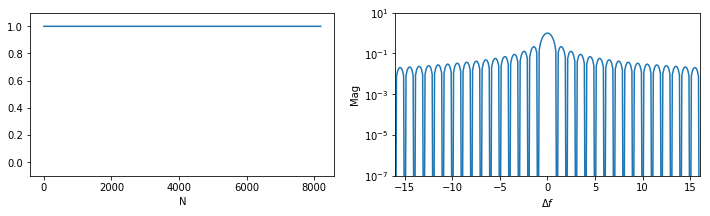### 汉宁窗

w(n) = 0.5(1-cos(\frac{2{\pi}n}{N-1})), n\in[0, N-1]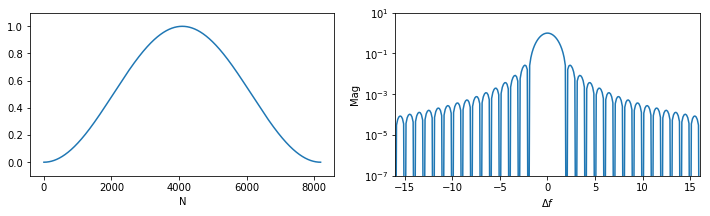### 平顶窗

w(n) = (1-1.93cos(\frac{2{\pi}n}{N-1})+1.29cos(\frac{4{\pi}n}{N-1})-0.388cos(\frac{6{\pi}n}{N-1})+0.0322cos(\frac{8{\pi}n}{N-1}))/4.634, n\in[0, N-1]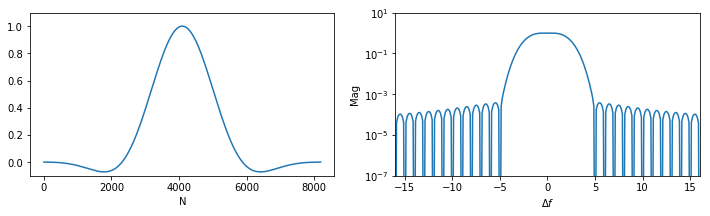### Blackman-Harris

w(n) = 0.35875 - 0.48829cos(\frac{2{\pi}n}{N-1})+0.14128cos(\frac{4{\pi}n}{N-1})-0.01168cos(\frac{6{\pi}n}{N-1})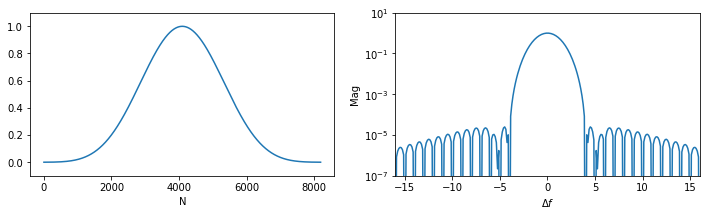### Kaiser-Bessel

w(n) = 0.40243 - 0.49804cos(\frac{2{\pi}n}{N-1})+0.09831cos(\frac{4{\pi}n}{N-1})-0.00122cos(\frac{6{\pi}n}{N-1})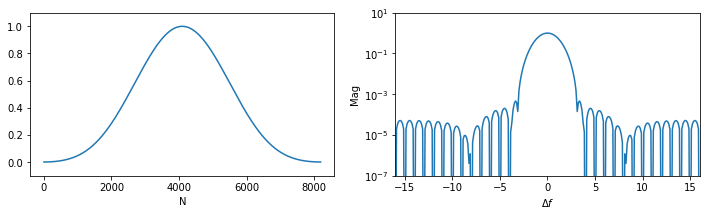### 窗函数的影响